# OEM 2019 Honda Civic Door Speaker Review

• Friday, Jul 31, 2020# Information

This speaker came from my very own 2019 Honda Civic Sport (non-Touring model). Standard stereo system (no upgrade). I was curious how this would measure so I measured it.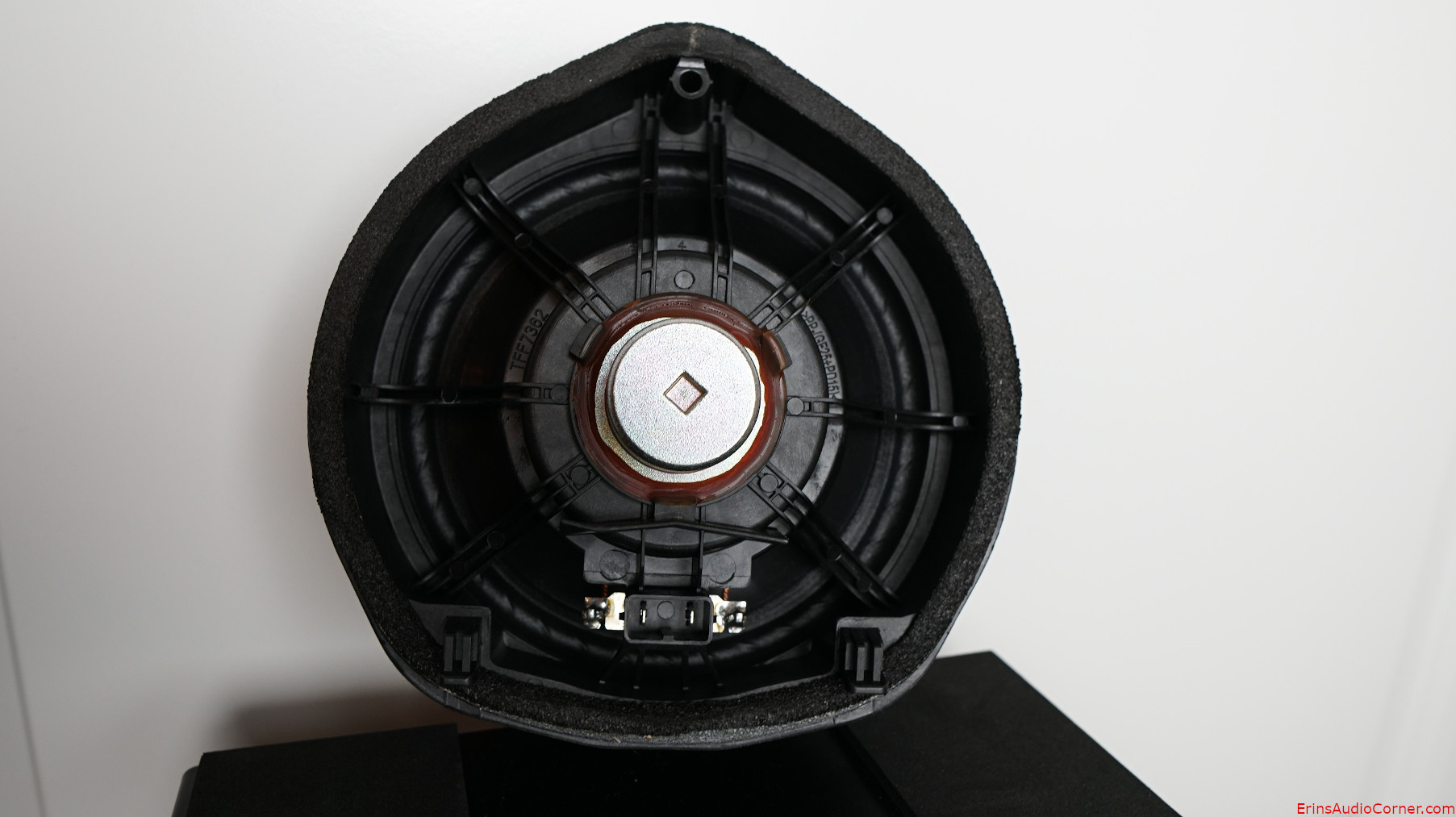# Test Results

While I would love to be able to confidently do so, I do not provide subjective analysis of raw drive units. Realistically, there is no easy way or meaningful way to do so. Drive units are parts of a whole. In order to test, subjectively, you would want to integrate it into a system and find what works best when mated to another driver (like a midwoofer to a tweeter, a midrange to a midwoofer, etc.). And to give it a fair shake it must be optimized in that inegration which would be a very arduous task to perform for every drive unit I review. It makes no sense to subjectively critique a single drive unit on its own because it may be used in a different configuration than someone else. One person may cross it with an LR2 HPF and LR4 LPF where another may use it in a completely different bandpass with completely different crossover and slopes. And if I were to try to create a speaker from it for the point of subjective analysis I am left with the same issue. The best implementation for a 6.5-inch midwoofer is different than it is for an 8-inch midwoofer. The data tells the story of performance on an apples-to-apples basis far better than someone's subjective imagination can.

For all the reasons listed above: What I provide here is objective-heavy analysis. However, I provide my own subjective experience and provide insight into what I believe might be positive or negative performance characteristics based on the objective data.

## Small Signal Testing (Thiele-Small Results)

Using Klippel’s Distortion Analyzer 2, Linear Lumped Parameter Measurement Module, Pro Driver Stand and provided Panasonic ANR12821 Laser along with Klippel’s Training 1 - Linear Lumped Parameter Measurement tutorial, I measured this drive unit’s impedance and small-signal parameters. Below are the results.

Electrical Parameters
Re 3.59 Ohm electrical voice coil resistance at DC
Le 0.039 mH frequency independent part of voice coil inductance
L2 0.224 mH para-inductance of voice coil
R2 1.99 Ohm electrical resistance due to eddy current losses
Cmes 1465.66 µF electrical capacitance representing moving mass
Lces 2.67 mH electrical inductance representing driver compliance
Res 14.44 Ohm resistance due to mechanical losses
fs 80.5 Hz driver resonance frequency
Mechanical Parameters
(using laser)
Mms 9.090 g mechanical mass of driver diaphragm assembly including air load and voice coil
Mmd (Sd) 7.321 g mechanical mass of voice coil and diaphragm without air load
Rms 0.430 kg/s mechanical resistance of total-driver losses
Cms 0.430 mm/N mechanical compliance of driver suspension
Kms 2.32 N/mm mechanical stiffness of driver suspension
Bl 2.490 N/A force factor (Bl product)
Lambda s 0.037 suspension creep factor
Loss factors
Qtp 2.132 total Q-factor considering all losses
Qms 10.698 mechanical Q-factor of driver in free air considering Rms only
Qes 2.658 electrical Q-factor of driver in free air considering Re only
Qts 2.129 total Q-factor considering Re and Rms only
Other Parameters
Vas 11.0632 l equivalent air volume of suspension
n0 0.209 % reference efficiency (2 pi-radiation using Re)
Lm 85.39 dB characteristic sound pressure level (SPL at 1m for 1W @ Re)
Lnom 85.87 dB nominal sensitivity (SPL at 1m for 1W @ Zn)
rmse Z 2.81 % root-mean-square fitting error of driver impedance Z(f)
rmse Hx 3.32 % root-mean-square fitting error of transfer function Hx (f)
Series resistor 0.00 Ohm resistance of series resistor
Sd 134.78 cm² diaphragm area

## Large Signal Modeling (Linear Xmax Results)

Using Klippel’s Distortion Analyzer 2, Large Signal Identification Module, Pro Driver Stand and provided Panasonic ANR12821 Laser along with Klippel’s Training 3 - Loudspeaker Nonlinearities tutorial, I measured the linear, nonlinear and thermal parameters of this drive unit.

### Nonlinearities

Traditionally, Xmax has been defined in one of the following ways:

• the physical overhang of the voice coil (height of the voice coil relative to height of the gap)
• 115% times the physical overhang above
• the point where displacement limit(s) is/are exceeded

The third option is where the Klippel LSI module comes in to play. It permits a more “apples to apples” approach of defining the displacement (Xmax) limits based on the XBL, XC, XL and Xd. The displacement limits XBL, XC, XL and Xd describe the limiting effect for the force factor Bl(x), compliance Cms(x), inductance Le(x) and Doppler effect, respectively, according to the threshold values Blmin, Cmin, Zmax and d2 used by the operator.

There are one of two sets of thresholds which can be used to define linear excursion:

1. Non-Subwoofer Drivers: The thresholds Blmin= 82 %, Cmin=75 %, Zmax=10 % and d2=10% generate for a two-tone-signal (f1=fs, f2=8.5fs) 10 % total harmonic distortion and 10 % intermodulation distortion.
2. Subwoofer Drivers: The thresholds Blmin= 70 %, Cmin=50 %, Zmax=17 % create 20 % total harmonic distortion which is becoming the standard for acceptable subwoofer distortion thresholds.

These parameters are defined in more detail in the (Klippel) papers:

• “AN04 – Measurement of Peak Displacement Xmax”
• “AN05 - Displacement Limits due to Driver Nonlinearities”
• “AN17 - Credibility of Nonlinear Parameters”
• “Prediction of Speaker Performance at High Amplitudes”
• “Assessment of Voice Coil Peak Displacement Xmax”
• “Assessing Large Signal Performance of Loudspeakers”

Below are the displacement limits’ results for this drive unit obtained from Klippel’s LSI module:

Displacement Limits
X Bl @ Bl min=82% 1.7 mm Displacement limit due to force factor variation
X C @ C min=75% 2.4 mm Displacement limit due to compliance variation
X L @ Z max=10 % >3.9 mm Displacement limit due to inductance variation
X d @ d2=10% 16.8 mm Displacement limit due to IM distortion (Doppler)
Asymmetry (IEC 62458)
Ak -9.36 % Stiffness asymmetry Ak(Xpeak)
Xsym 0.49 mm Symmetry point of Bl(x) at maximal excursion

Per the above table, this drive unit’s linear excursion is limited to 1.7mm due to exceeding the Bl displacement limit of 82% for the distortion limit of 10%.

We can break the above information down further. The below text is written by Patrick Turnmire of Red Rock Acoustics and used with his permission, substituting data from this drive unit’s test results where applicable.

#### Large Signal Modeling

At higher amplitudes, loudspeakers produce substantial distortion in the output signal, generated by nonlinear ties inherent in the transducer. The dominant nonlinear distortions are predictable and are closely related with the general principle, particular design, material properties and assembling techniques of the loudspeaker. The Klippel Distortion Analyzer combines nonlinear measurement techniques with computer simulation to explain the generation of the nonlinear distortions, to identify their physical causes and to give suggestion for constructional improvements. Better insight into the nonlinear mechanisms makes it possible to further optimize the transducer in respect with sound quality, weight, size and cost.

#### Nonlinear Characteristics

The dominant nonlinearities are modelled by variable parameters such as

Bl(x) instantaneous electro-dynamic coupling factor (force factor of the motor) defined by the integral of the magnetic flux density B over voice coil length l as a function of displacement
KMS(x) mechanical stiffness of driver suspension a function of displacement
LE(i) voice coil inductance as a function of input current (describes nonlinear permeability of the iron path)
LE(x) voice coil inductance as a function of displacement

#### Nonlinear Parameters

Bl change with excursionThe electrodynamic coupling factor, also called Bl-product or force factor Bl(x), is defined by the integral of the magnetic flux density B over voice coil length l and translates current into force. In traditional modeling this parameter is assumed to be constant. The force factor Bl(0) at the rest position corresponds with the Bl-product used in linear modeling. The red curve displays Bl over the entire displacement range covered during the measurement. You see the typical decay of Bl when the voice coil moves out of the gap. At the end of the measurement, the black curve shows the confidential range (interval where the voice coil displacement in this range occurred 99% of the measurement time). During the measurement, the black curve shows the current working range. The dashed curve displays Bl(x) mirrored at the rest position of the voice coil – this way, asymmetries can be quickly identified. Since a laser was connected during the measurement, a coil in / coil out marker is displayed on the bottom left / bottom right.

Suspension Stiffness change with excursion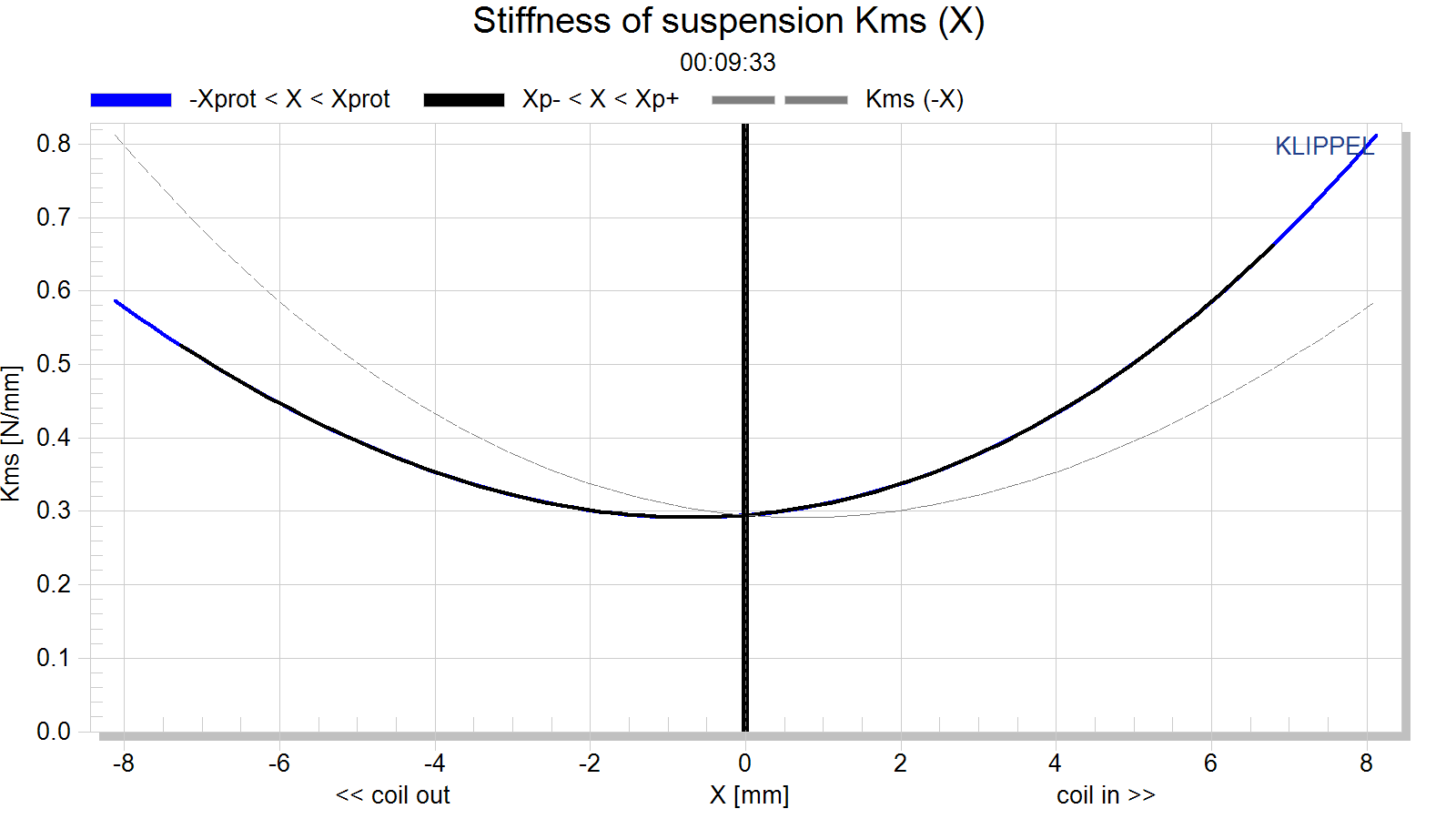The stiffness KMS(x) describes the mechanical properties of the suspension. Its inverse is the compliance CMS(x).

Inductance change with excursion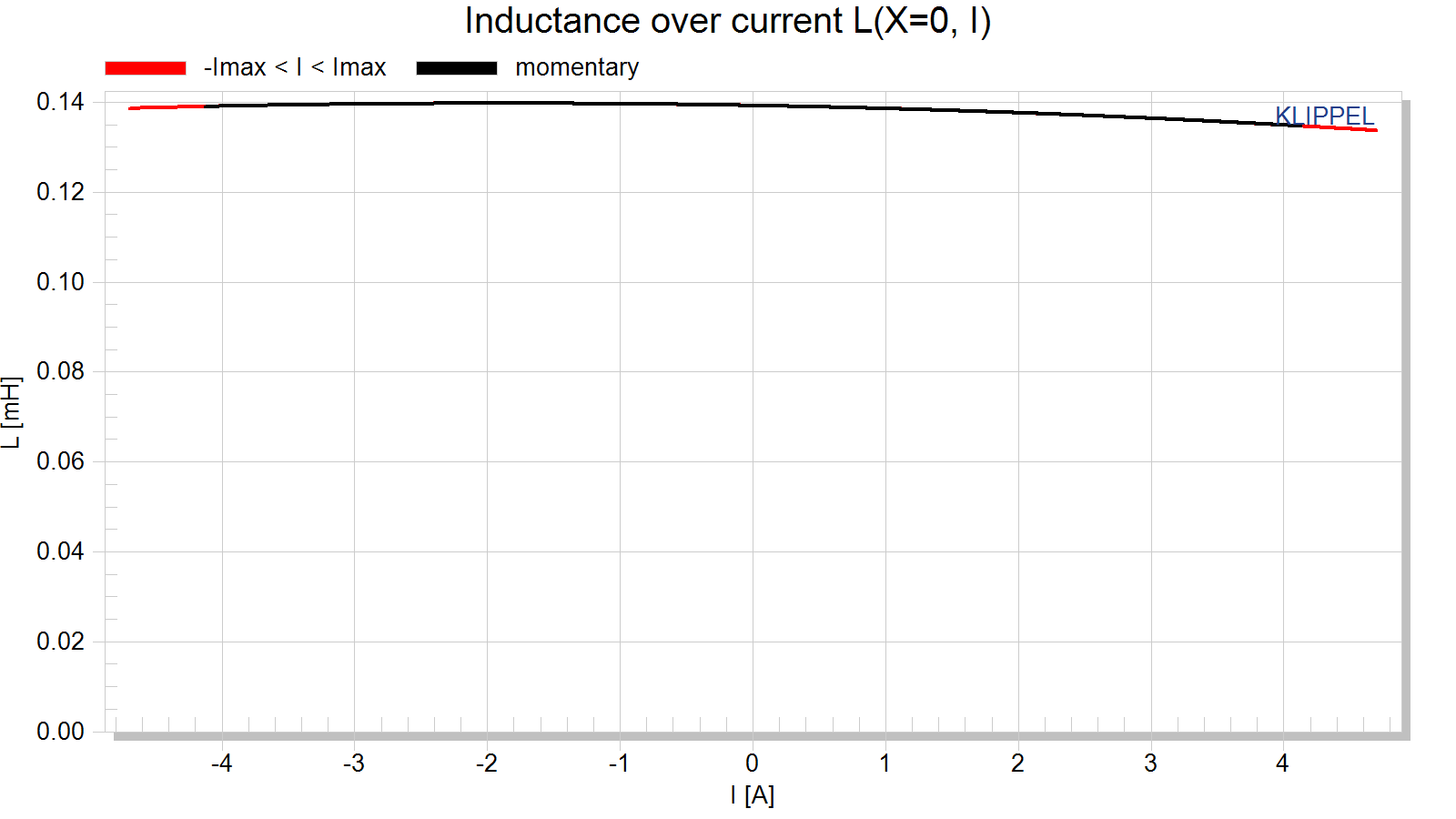The inductance components Le (x) and Bl(i) of most drivers have a strong asymmetric characteristic. If the voice coil moves towards the back plate the inductance usually increases since the magnetic field generated by the current in the voice coil has a lower magnetic resistance due to the shorter air path. The nonlinear inductance Le(x) has two nonlinear effects. First the variation of the electrical impedance with voice coil displacement x affects the input current of the driver. Here the nonlinear source of distortion is the multiplication of displacement and current. The second effect is the generation of a reluctance force which may be interpreted as an electromagnetic motor force proportional to the squared input current.

The flux modulation Bl(i) has two effects too. On the electrical side the back EMF Bl(i)*v produces nonlinear distortion due to the multiplication of current i and velocity v. On the mechanical side the driving force F = Bl(i)*i comprises a nonlinear term due to the squared current i. This force produces similar effects as the variable term Le(x).

Fs shift with excursion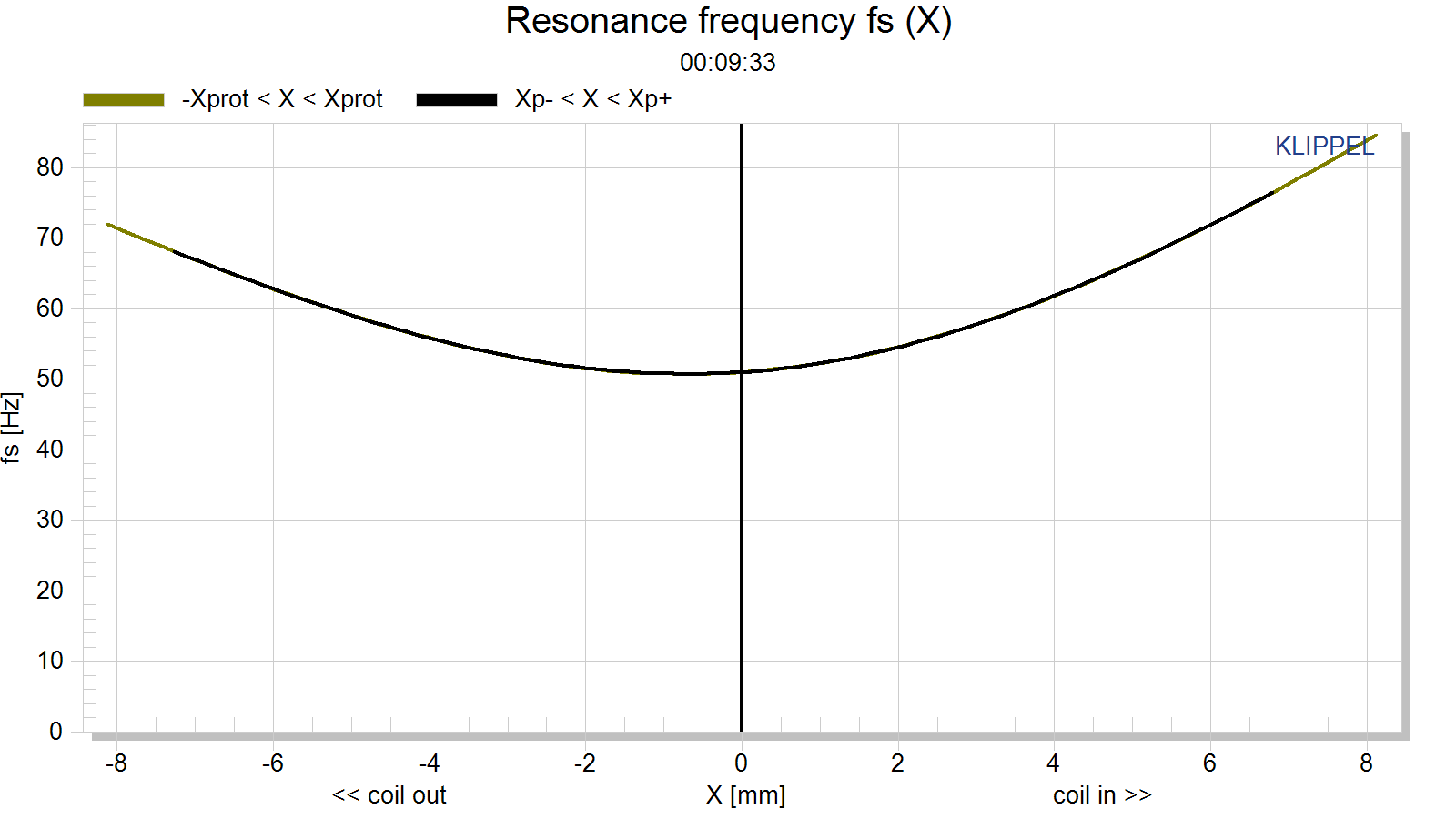Qts change with excursion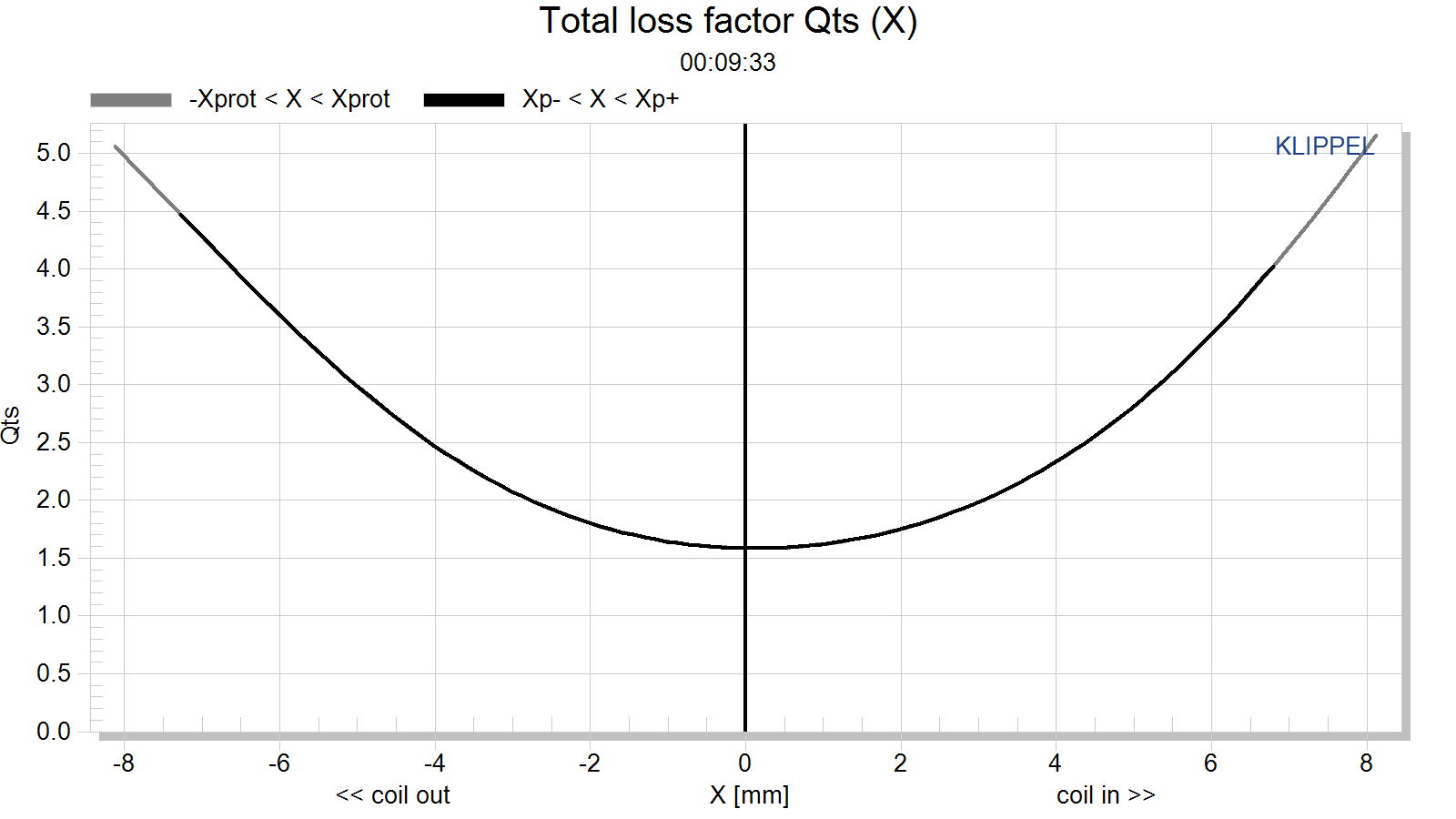#### Asymmetrical Nonlinearities

Asymmetrical nonlinearities produce not only second- and higher-order distortions but also a dc-part in the displacement by rectifying low frequency components. For an asymmetric stiffness characteristic the dc-components moves the voice coil for any excitation signal in the direction of the stiffness minimum. For an asymmetric force factor characteristic the dc-component depends on the frequency of the excitation signal. A sinusoidal tone below resonance (f<fS) would generate or force moving the voice coil always in the force factor maximum. This effect is most welcome for stabilizing voice coil position. However, above the resonance frequency (f>fS) would generate a dc-component moving the voice coil in the force factor minimum and may cause severe stability problems. For an asymmetric inductance characteristic the dc-component moves the voice coil for any excitation signal in the direction of the inductance maximum. Please note that the dynamically generated DC-components cause interactions between the driver nonlinearities. An optimal rest position of the coil in the gap may be destroyed by an asymmetric compliance or inductance characteristic at higher amplitudes. The module Large Signal Simulation (SIM) allows systematic investigation of the complicated behavior.

Bl symmetry xb(x)

This curve shows the symmetry point in the nonlinear Bl-curve where a negative and positive displacement x=xpeak will produce the same force factor Bl(xb(x) + x) = Bl(xb(x) – x).

If the shift xb(x) is independent on the displacement amplitude x then the force factor asymmetry is caused by an offset of the voice coil position and can be simply compensated.

If the optimal shift xb(x) varies with the displacement amplitude x then the force factor asymmetry is caused by an asymmetrical geometry of the magnetic field and cannot completely be compensated by coil shifting.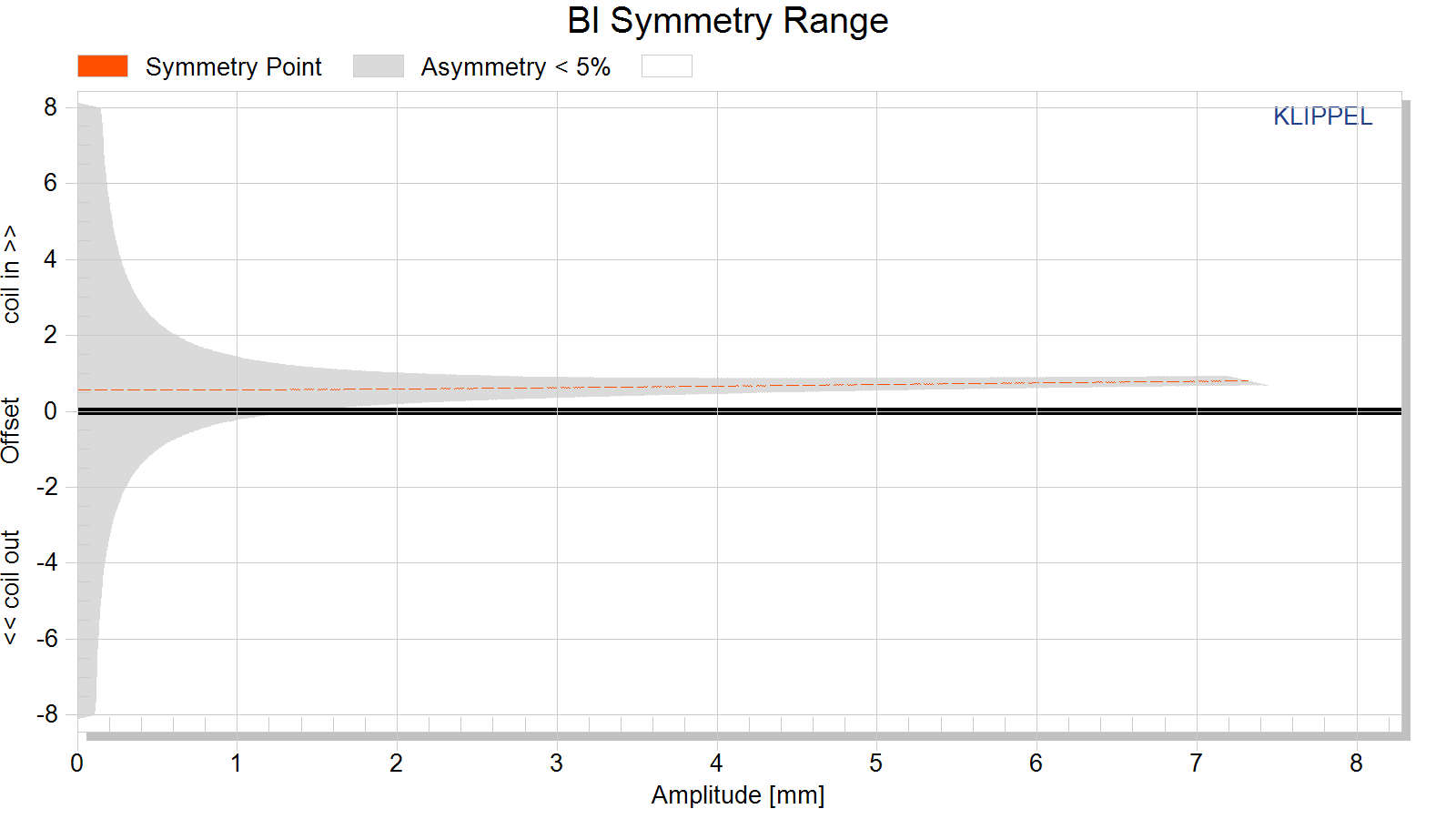Kms Symmetry xc(x)

This curve shows the symmetry point in the nonlinear compliance curve where a negative and positive displacement x=xpeak will produce the same compliance value kms(xc(x) + x) = kms(xc(x) – x).

A high value of the symmetry point xc(x) at small displacement amplitudes x » 0 indicates that the rest position does not agree with the minimum of the stiffness characteristic. This may be caused by an asymmetry in the geometry of the spider (cup form) or surround (half wave). A high value of the symmetry point xc(x) at maximal displacement x» xmax may be caused by asymmetric limiting of the surround.#### Parameters at the Rest Position

For accurate system modelling “Large + Cold” parameters are preferable to “Small Signal” parameters because they more closely reflect the parameters in their typical operating range.

Symbol Large + Warm Large + Cold Small Signal Unit Comment
Note: for accurate small signal parameters, use LPM module
———————– ——— ——— ——— —— ————————————————————————————————————–
Delta Tv [referenced] 95.5 0 K increase of voice coil temperature during the measurement referenced to imported Re(Delta Tv=0K)
Delta Tv = Tv-Ta 82.5 0 0 K increase of voice coil temperature during the measurement
Xprot 4.5 4.5 1.0 mm maximal voice coil excursion (limited by protection system)
Re (Tv) 4.89 3.72 3.72 Ohm voice coil resistance considering increase of voice coil temperature Tv
Le (X=0) 0.15 0.15 0.14 mH voice coil inductance at the rest position of the voice coil
L2 (X=0) 0.28 0.28 0.25 mH para-inductance at the rest position due to the effect of eddy current
R2 (X=0) 0.62 0.62 0.60 Ohm resistance at the rest position due to eddy currents
Cmes (X=0) 2137 2137 1665 µF electrical capacitance representing moving mass
Lces (X=0) 4.05 4.05 3.46 mH electrical inductance at the rest position representing driver compliance
Res (X=0) 6.39 6.39 6.80 Ohm resistance at the rest position due to mechanical losses
Qms (X=0, Tv) 4.64 4.64 4.72 mechanical Q-factor considering the mechanical system only
Qes (Tv) 2.70 2.06 2.58 electrical Q-factor considering Re (Tv) only
Qts (X=0, Tv) 1.71 1.43 1.67 total Q-factor considering Re (Tv) and Rms only
fs 54.1 54.1 66.3 Hz driver resonance frequency
Mms 10.091 10.091 g (calculated from imported Bl) mechanical mass of driver diaphragm assembly including voice-coil and air load
Rms (X=0) 0.739 0.739 0.912 kg/s mechanical resistance of total-driver losses
Cms (X=0) 0.86 0.86 0.56 mm/N mechanical compliance of driver suspension at the rest position
Kms (X=0) 1.16 1.16 1.79 N/mm mechanical stiffness of driver suspension at the rest position
Bl (X=0) 2.49 2.49 2.49 N/A (imported) force factor at the rest position (Bl product)
Vas 21.9881 21.9881 14.2946 l equivalent air volume of suspension
N0 0.123 0.162 0.155 % reference efficiency (2Pi-sr radiation using Re)
Lm 83.1 84.2 84.0 dB characteristic sound pressure level
Sd 134.78 134.78 134.78 cm² diaphragm area

## Power vs SPL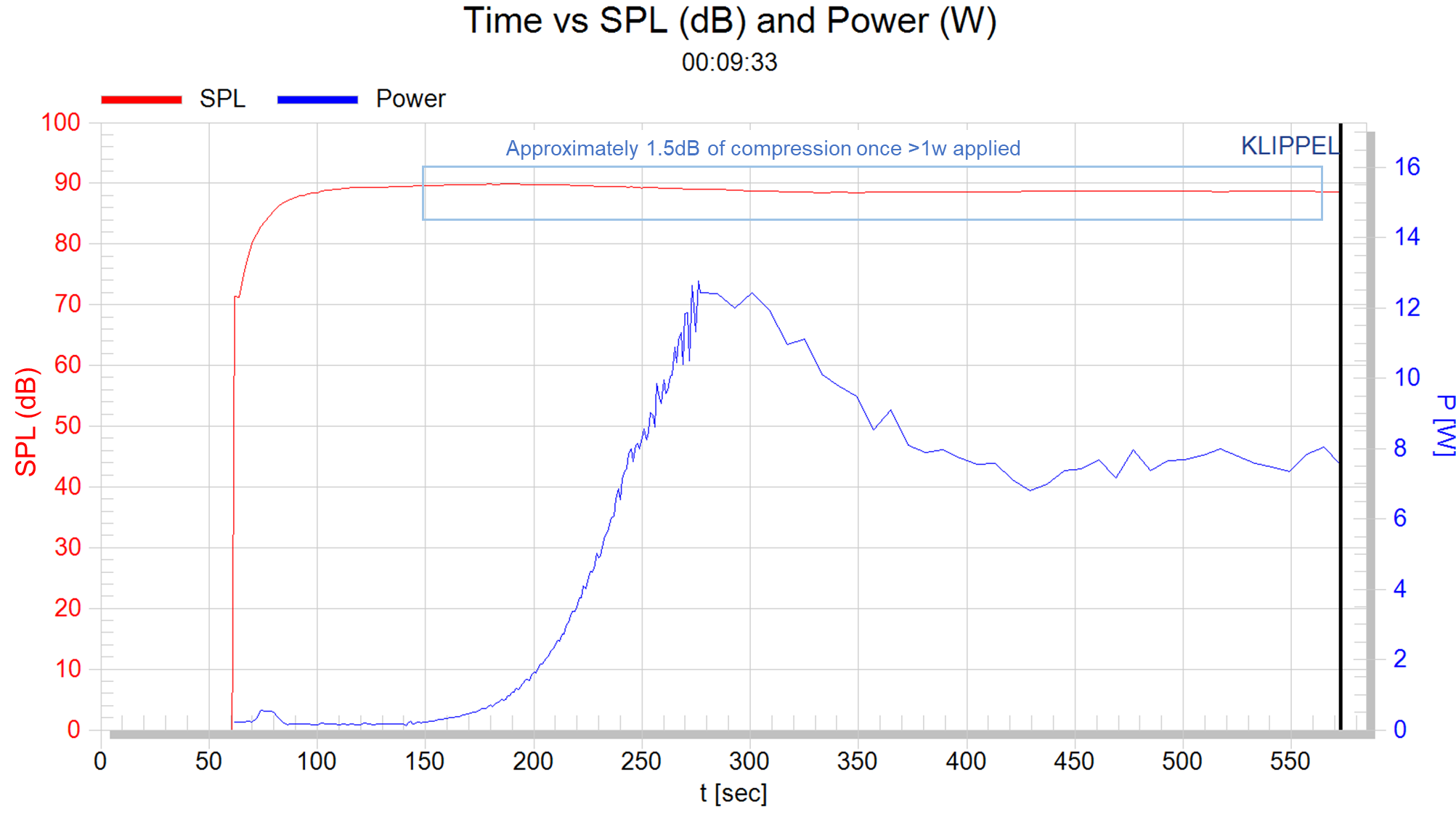# Bottom Line

• Well, as you’d expect, there’s not much linear throw here. Roughly about 1.7mm one-way, limited by the motor force.
• Mechanical excursion is around 4mm one-way.
• Surprisingly, there does seem to be some form of inductance control.
• The suspension is very tight with a Qts of 1.82. Combined with an Fs of 67Hz. The very high Qts creates a sharp peak in output at Fs followed by a sharp rolloff. This means there will be a very, very punchy midbass sound but with nothing around it to give it fullness. Additionally, the speaker is less likely to bottom out because the suspension won’t permit the speaker to move easily and the motor force is so low (at about 1.32) that it wouldn’t be able to drive the suspension past mechanical excursion.
• If you look at the Excursion vs Fs graphic you can see the ‘rest’ position for Fs is approximately 51Hz. At 3mm forward, it has increased to about 60Hz and by 6mm forward it has increased to 70Hz. A similarly sharp increase in value is observed in Qts.
• Once the speaker has received more than about 1 watt the output dips from about 90dB to about 88.5dB. A loss of 1.5dB due to thermal compression. In other words, this speaker’s voice coil is being saturated with heat and output is lost.

# Contribute

If you like what you see here and want to help me keep it going, you can donate via the PayPal Contribute button at the bottom of each page. If you can help by chipping in, I would truly appreciate it.